## 范畴和态射• 集合范畴由所有集合和它们之间的映射(关系)组成；
• 向量空间范畴由所有向量空间和它们之间的线性映射组成；
• 群范畴由群和它们之间的关系(群同态)组成；
• 拓扑空间范畴由拓扑空间和它们之间的关系(连续映射)组成；

• 范畴论是一门关于箭头(态射)的科学，箭头是用来连接两个点(对象)的。
• 范畴论是一门元关系(meta-relation)学科。
• 不同领域的问题，如果具有相似的关系，在范畴论便可以统领(套路)。
• 借助范畴论的各种套路，可以把某个领域的问题转化为其它领域的问题(函子)。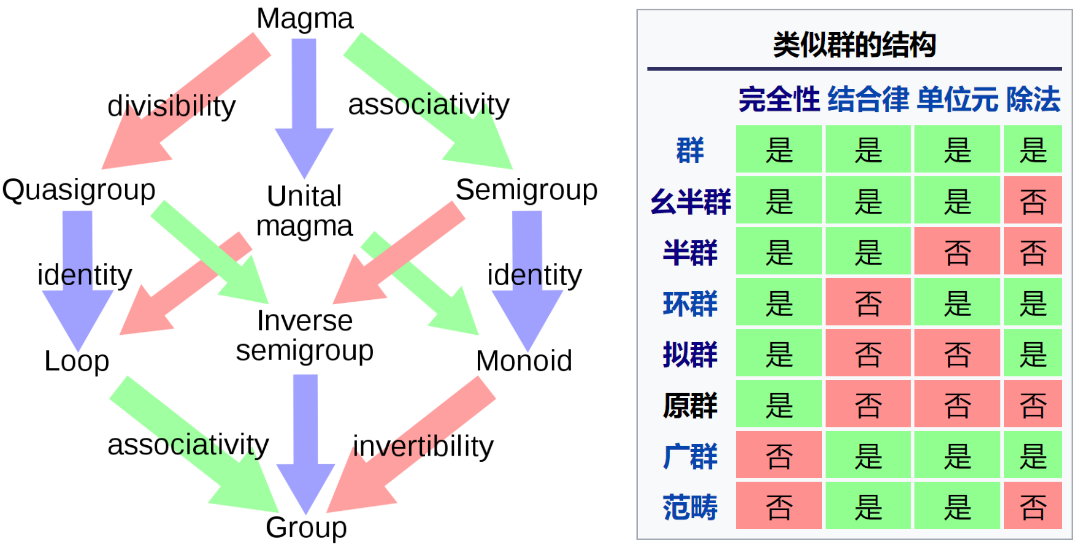• 单态射，
• 满态射，
• 同构，
• 自同构，
• 自同态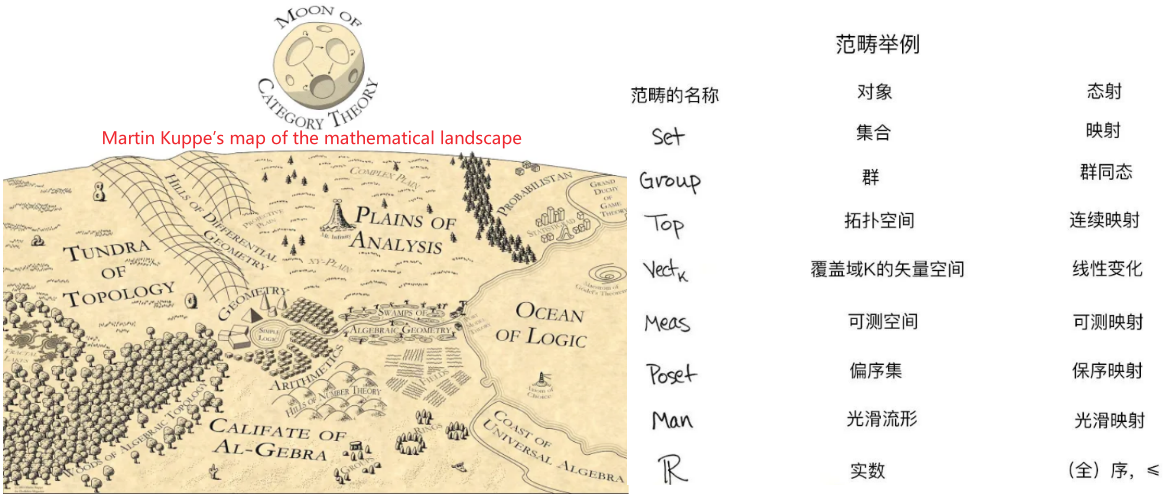• 【马丁·库佩的数学地图】范畴论高高悬挂在天空，提供了整个地图的缩略图。它让我们能够看到在地面看不到的各个领域之间的关系，证明看似不相关的数学领域并不是完全不同。当你想解决某个领域(比如说拓扑)中的问题，但没有合适的工具可以使用时，这就变得非常有用。通过将问题转移到不同领域(比如群论)，就能让你换个角度看问题，说不定还能发现新的工具，让问题变得更容易解决。事实上，范畴论就是这样产生的。它诞生于20世纪40年代，背景是人们试图用更简单的代数方法来解决一个困难的拓扑问题。参考Mathematics: Measuring x laziness²(视频)
• 范畴论的一个主要特点是它剥离了很多细节：它并不具体关心集合中的某个元素，或者某个群是否可解，或者某个拓扑空间是否有可列基。剥离细节的一个好处是，我们的注意力从单个对象上转移开，转向它们之间存在的关系——态射，关系就是一切
• 一个数学对象完全由它与所有其他对象的关系决定。换句话说，当且仅当两个对象以同样方式与范畴中的每个对象相关时，两个对象本质上是不可区分的。

## 旧笔记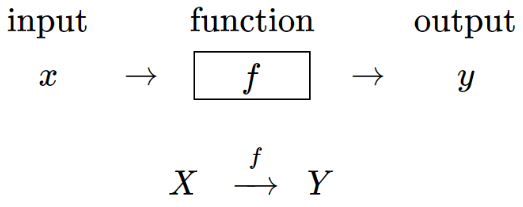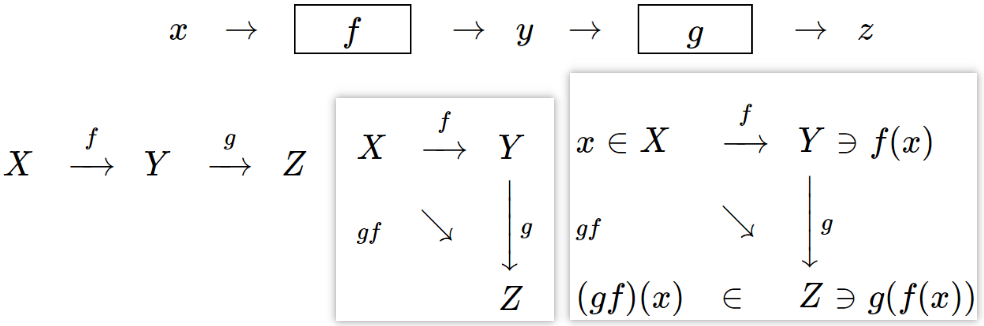上面右下侧的两种表示方式，给出了新的函数$$gf$$，completes the diagram。从$$X$$到$$Z$$，两条路线都是殊途同归的，即$$g(f(x))=(g f)(x)$$。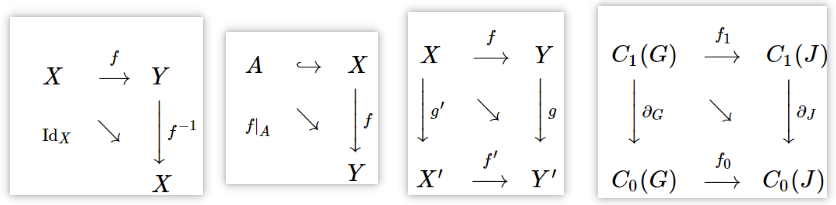• 用到了函数$$f$$的逆运算$$f^{-1}$$；
• $$A$$是$$X$$的子集$$A \subset X$$，交换图中$$\displaystyle\hookrightarrow$$表示，最常见的子集，比如$$A=\operatorname{ker} f$$；
• square shape的交换图，$$g f=f^{\prime} g^{\prime}$$，也就是说，先垂直往下走，再往右走，等价于先往右走，再往下走，也等价于直积走对角线。Both give you the diagonal function! This identity is the reason why it makes sense to call such a diagram "commutative".感觉在织布
• Algebraic continuity condition，表示为$$\partial_J f_1(e)=f_0\left(\partial_G e\right)$$。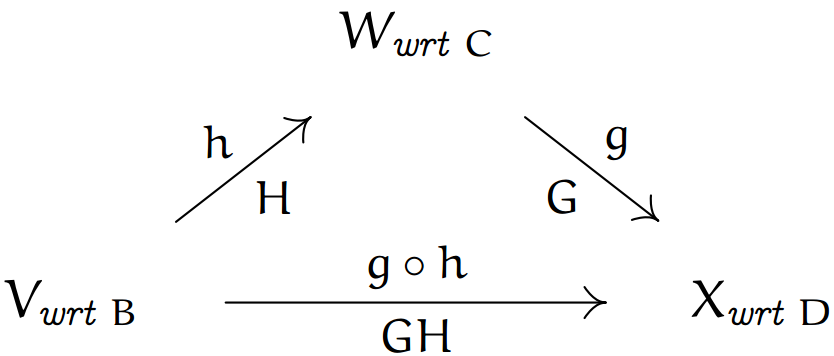上图中的wrt表示with respect to，$$V_{\text {wrt } \mathrm{B}}$$表示将$$V$$空间写成以$$\mathrm{B}$$为基底的形式。或者等价地表示为$$\vec{v} \stackrel{g \circ h}{\longmapsto} g(h(\vec{v})) \quad \vec{v} \stackrel{h}{\longmapsto} h(\vec{v}) \stackrel{g}{\longmapsto} g(h(\vec{v}))$$注意：映射和基的选取无关，但是表示映射的矩阵和基的选取有关，即描述同一件事可以用不同的表达方式，但是殊途同归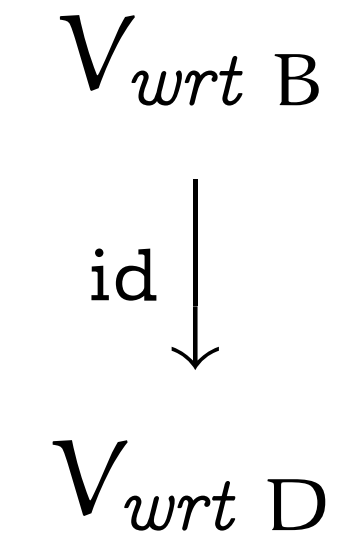• 小写字母表示映射，大写字母表示在domain space的基确定的情况下，该映射的矩阵表示；
• $$\operatorname{Rep}_{\mathrm{B}}(\vec{v})$$为向量$$\vec{v}$$在基$$\mathrm{B}$$下的坐标表示，或者说该向量在该基下各基向量方向的投影大小组成的一组数；
• $$\operatorname{Rep}_{\mathrm{B}, \mathrm{D}}(h)$$，即存在一个映射$$h$$，domain space的基为$$\mathrm{B}$$，codomain space的基为$$\mathrm{D}$$，至于两个space是不是同一个space或者说维度上有什么关系，我们并不关心。$$\mathrm{D}\cdot\operatorname{Rep}_{\mathrm{B}, \mathrm{D}}(h)$$表示的codomain space的基(列向量)组成的矩阵$$\mathrm{D}$$乘以$$\operatorname{Rep}_{\mathrm{B}, \mathrm{D}}(h)$$，即为domain space的基通过映射$$h$$作用后得到的codomain space中的向量组(绝对结果，和基无关)，这个可以看作是自然基状态下的表示(更容易理解)，因为映射就是映射，和基的选取无关。